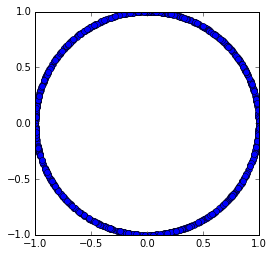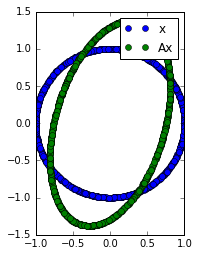# Matrix norms¶

In :
import numpy as np
import numpy.linalg as la
import matplotlib.pyplot as pt


Here's a matrix of which we're trying to compute the norm:

In :
n = 2
A = np.random.randn(n, n)
A

Out:
array([[-0.12603194,  0.74240991],
[ 0.07209953, -0.91593647]])

Recall:

$$||A||=\max_{\|x\|=1} \|Ax\|,$$

where the vector norm must be specified, and the value of the matrix norm $\|A\|$ depends on the choice of vector norm.

For instance, for the $p$-norms, we often write:

$$||A||_2=\max_{\|x\|=1} \|Ax\|_2,$$

and similarly for different values of $p$.

We can approximate this by just producing very many random vectors and evaluating the formula:

In :
xs = np.random.randn(n, 1000)


First, we need to bring all those vectors to have norm 1. First, compute the norms:

In :
p = 2
norm_xs = np.sum(np.abs(xs)**p, axis=0)**(1/p)
norm_xs.shape

Out:
(1000,)

Then, divide by the norms and assign to normalized_xs:

Then check the norm of a randomly chosen vector.

In :
normalized_xs = xs/norm_xs
la.norm(normalized_xs[:, 316], p)

Out:
1.0

Let's take a look:

In :
pt.plot(normalized_xs, normalized_xs, "o")
pt.gca().set_aspect("equal")Now apply $A$ to these normalized vectors:

In :
A_nxs = A.dot(normalized_xs)


Let's take a look again:

In :
pt.plot(normalized_xs, normalized_xs, "o", label="x")
pt.plot(A_nxs, A_nxs, "o", label="Ax")
pt.legend()
pt.gca().set_aspect("equal")Next, compute norms of the $Ax$ vectors:

In :
norm_Axs = np.sum(np.abs(A_nxs)**p, axis=0)**(1/p)
norm_Axs.shape

Out:
(1000,)

What's the biggest one?

In :
np.max(norm_Axs)

Out:
1.4175372998707851

Compare that with what numpy thinks the matrix norm is:

In :
la.norm(A, p)

Out:
1.4175373681672399